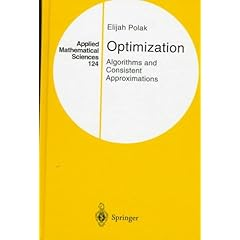Optimization: Algorithms and Consistent Approximations covers algorithms and discretization procedures for the solution of nonlinear programming, semi-infinite optimization and optimal control problems. Readers will find of particular interest the exhaustive modern treatment of optimality conditions and algorithms for min-max problems, as well as the newly developed theory of consistent approximations and the treatment of semi-infinite optimization and optimal control problems in this framework. This book presents the first treatment of optimization algorithms for optimal control problems with state-trajectory and control constraints, and fully accounts for all the approximations that one must make in their solution. It is also the first to make use of the concepts of epi-convergence and optimality functions in the construction of consistent approximations to infinite dimensional problems